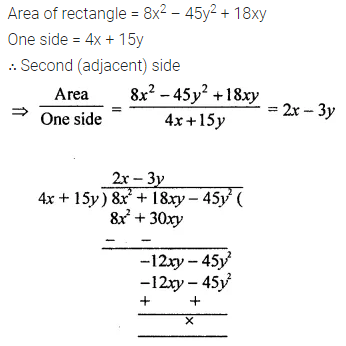## ML Aggarwal Class 8 Solutions for ICSE Maths Chapter 10 Algebraic Expressions and Identities Ex 10.4

Question 1.
Divide:
(i) – 39pq2r5 by – 24p3q3r
(ii) –$$\frac{3}{4}$$a2b3 by $$\frac{6}{7}$$a3b2
Solution: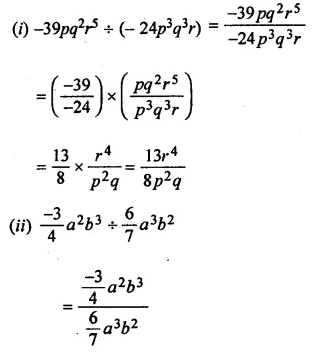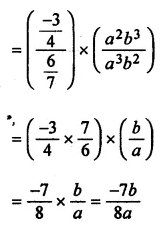Question 2.
Divide:
(i) 9x4 – 8x3 – 12x + 3 by 3x
(ii) 14p2q3 – 32p3q2 + 15pq2 – 22p + 18q by – 2p2q.
Solution: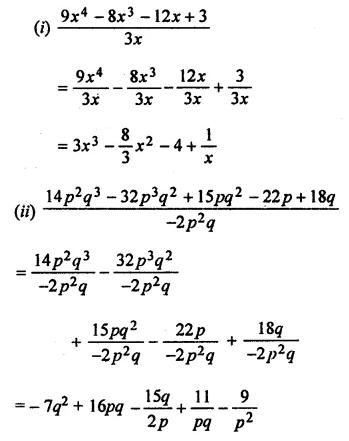Question 3.
Divide:
(i) 6x2 + 13x + 5 by 2x + 1
(ii) 1 + y3 by 1 + y
(iii) 5 + x – 2x2 by x + 1
(iv) x3 – 6x2 + 12x – 8 by x – 2
Solution: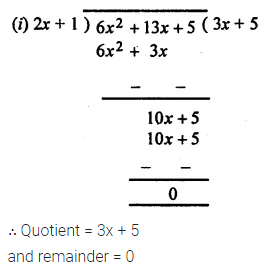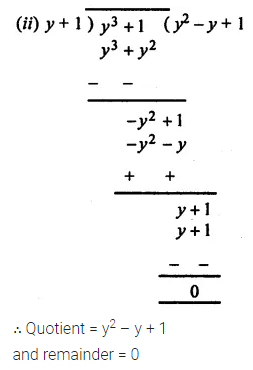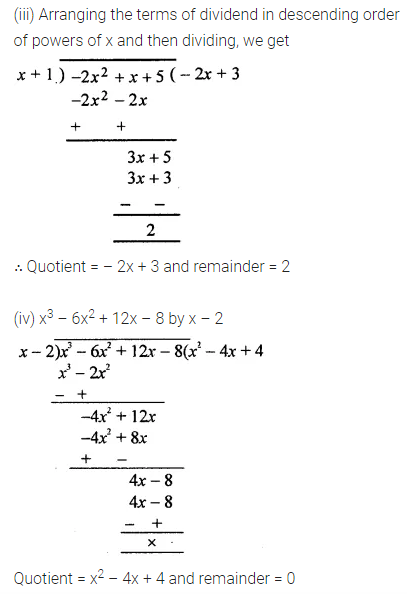Question 4.
Divide:
(i) 6x3 + x2 – 26x – 25 by 3x – 7
(ii) m3 – 6m2 + 7 by m – 1
Solution: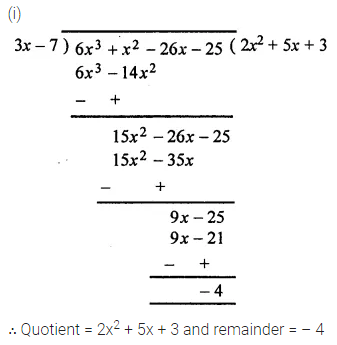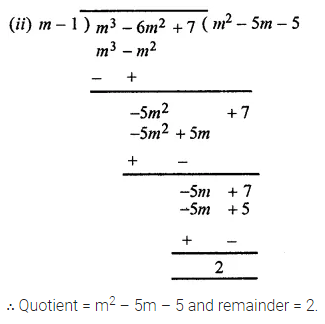Question 5.
Divide:
(i) a3 + 2a2 + 2a + 1 by + a2 + a + 1
(ii) 12x3 – 17x2 + 26x – 18 by 3x2 – 2x + 5
Solution:Question 6.
If the area of a rectangle is 8x2 – 45y2 + 18xy and one of its sides is 4x + 15y, find the length of the adjacent side.
Solution: